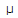# Electronics - Capacitors - Discussion

### Discussion :: Capacitors - General Questions (Q.No.38)

38.

What is the value of a capacitor labeled 102?

 [A]. 10,000 pF [B]. 1000 pF [C]. 1000F [D]. 10,000F

Explanation:

No answer description available for this question.

 Ashok said: (Sep 4, 2011) There is a three digit code printed on a ceramic capacitor specifying its value. The first two digits are the two significant figures and the third digit is a base 10 multiplier. The value is given in picofarads (pF). A letter suffix indicates the tolerance. C = ± 0.25 pF M = ±20% D = ± 0.5 pF P = +100 -0% J = ± 5% Y = -20 +50% K = ±10% Z = -20 + 80% Example: a label of "104K" indicates 10x10^4 pF = 100,000 pF = 100 nF = 0.1 µF ±10% Now the ans of this question is: a label of '102' indicates 10x10^2 pF = 1000 pF.

 Seema said: (Dec 2, 2011) Please can anyone explain more simply.

 Athul said: (Nov 19, 2012) 102 means 10*10^2 pf. If its 103 then 10*10^3 pf.

 Ram said: (Dec 23, 2012) If its 99, then ?

 Sunil said: (Sep 2, 2014) Please anyone answer. How can we get capacitive value of any 2,3 digit no? If any no except 3dig like 101, 102...please.

 Nikhil said: (Sep 18, 2014) Often the capacitor will simply be marked with a two digit number printed on the body such as "10" for example. This indicates that it is a 10pF capacitor. However you may find some capacitors marked "10n" and this capacitor will have a value of 10nF (ie 10,000pF), this is sometimes seen on polystyrene types and some resin dipped ceramics. To make matters rather more confusing, when we eventually arrive home with a plastic bag full of components keen to construct a circuit we find that many capacitors are marked with a three digit code such as "103" or "104" and some others have a three digit code plus a letter on the end such as "101K" or "102K". The capacitors marked with three digits are similar to resistors in that the first two digits represent the value in pF (as above) and the third digit is the multiplier with a letter to indicate the Tolerance. So "100" would be 10pF multiplied by zero i.e. 10pF. "103" is 10pF multiplied by 1000 ie 10,000pF or to put is another way 0.01 microfarads. "471K" would be a 470pF capacitor with a 10% Tolerance.